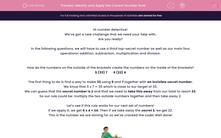# Identify and Apply the Correct Number Rule

In this worksheet, students will identify a rule which is used to make a number and apply this rule to find a missing number.Key stage:  KS 2

Curriculum topic:   Verbal Reasoning

Curriculum subtopic:   Number Logic

Difficulty level:#### Worksheet Overview

Hi number detective!

We’ve got a new challenge that we need your help with.

In the following questions, we will have to use a third top-secret number as well as our main four operations: addition, subtraction, multiplication and division.

How do the numbers on the outside of the brackets create the numbers on the inside of the brackets?

5 (33) 7        6 (22) 4

The first thing to do is find a way to make 33 using 5 and 7 together with an invisible secret number.

We know that 5 x 7 = 35 which is close to our target of 33.

We can guess that the secret number is 2 and that we need to take this away from our total to reach 33.

So our rule could be: multiply the two outside numbers together and then take away 2.

Let’s see if this rule works for our next set of numbers!

If we apply it, we get 6 x 4 = 24. Then if we take away the secret 2, we get 22.

This is the number we are aiming for so we’ve cracked the code! Well done!For the next question, we need to crack the code and then use the rule to work out the missing number.

3 (11) 5        4 (16) 9        2 (_) 7

First, we need to find a way to make 11 using 3 and 5 together with an invisible secret number.

We could try 3 x 5 = 15 and then take away the secret number of 4 to get 11.

So our rule could be: multiply the two outside numbers and then take away 4.

Let’s see if this rule works for the next set of numbers: 4 x 9 = 36 and 36 - 4 = 32.

Oh dear! We didn’t find the right rule this time which means we need to look for another.

We could try 3 + 5 = 8 and then add a secret number of 3.

So our rule could be: add the two outside numbers and then add an extra 3.

Let’s try that for the next set of numbers: 4 + 9 = 13 and then add the extra 3 is 16.

Jackpot! We’ve found the right rule!

Now let’s use this rule to work out the missing number in the final set of numbers.

2 + 7 = 9 and then add the extra 3 gives us 12.

So the correct answer is 12.Pssstt! Here's a handy hint to help you reach superstar status: look out for the secret number and don't worry if the first rule you find doesn't work, just stay calm and try another one!

It’s now your turn to put your skills to the test and act as a number detective, hunting down those missing numbers!

### What is EdPlace?

We're your National Curriculum aligned online education content provider helping each child succeed in English, maths and science from year 1 to GCSE. With an EdPlace account you’ll be able to track and measure progress, helping each child achieve their best. We build confidence and attainment by personalising each child’s learning at a level that suits them.

Get started